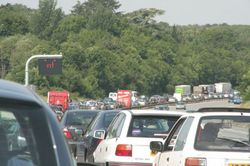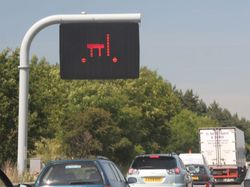### jammin'

we got stuck in a massive queue on the motorway yesterday heading for the Thames Path.interestingly they had some fancy signs up showing mathematical symbols. i asked jane about it and she said it was probably a formula for the queue length which i thought was inspired.so what is 'pi' multiplied by ' l' ? pi is obviously this, but what is the symbol 'l' representing in this formula ?

i wondered if the formula was a way of calculating the time (in minutes) it will take to get through the queue: ie it's 3.14 times the length ('l') of the queue in miles.

that would be about right as the queue was about 10 miles long and it took us over half an hour to get through it.

any other ideas ?